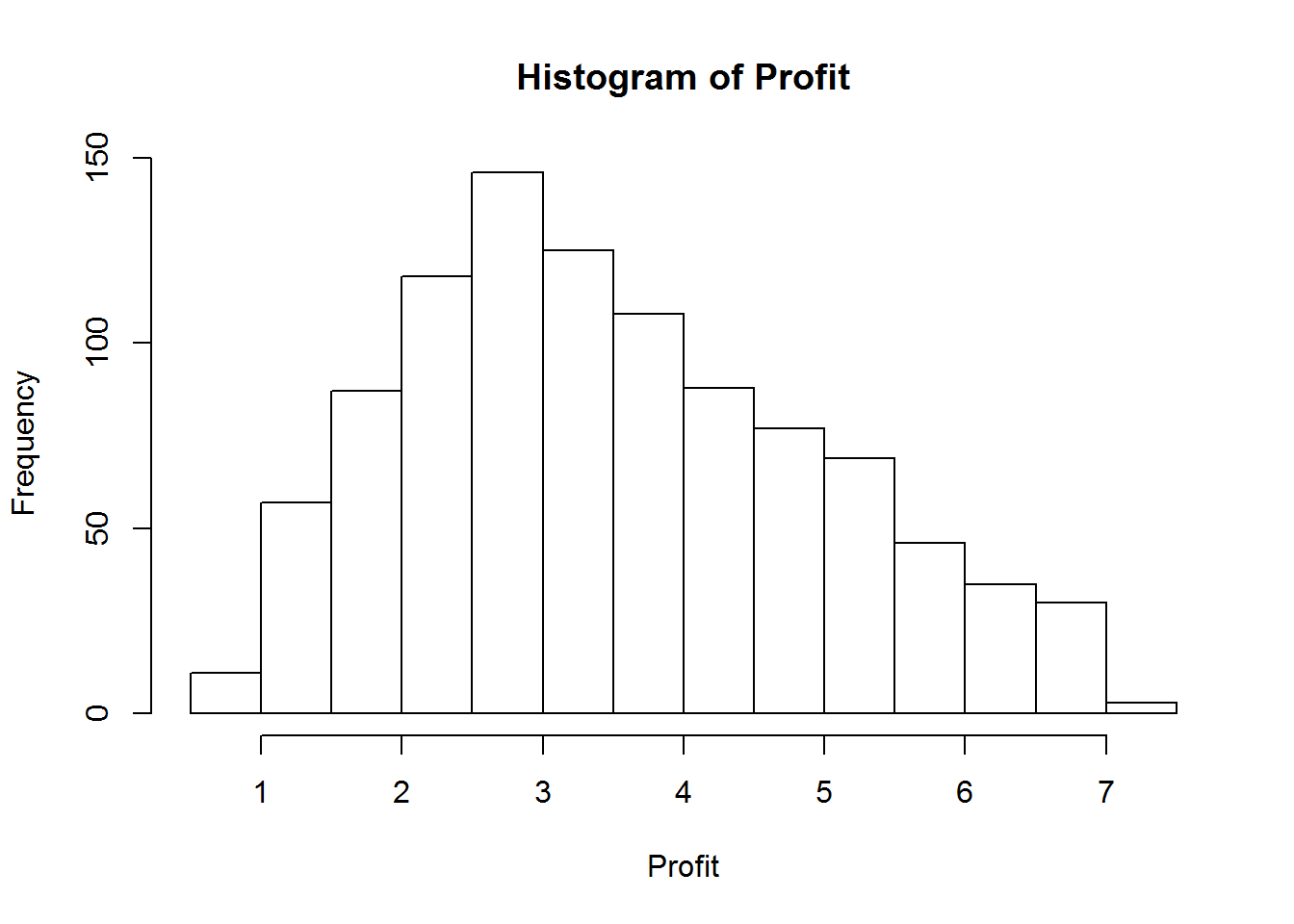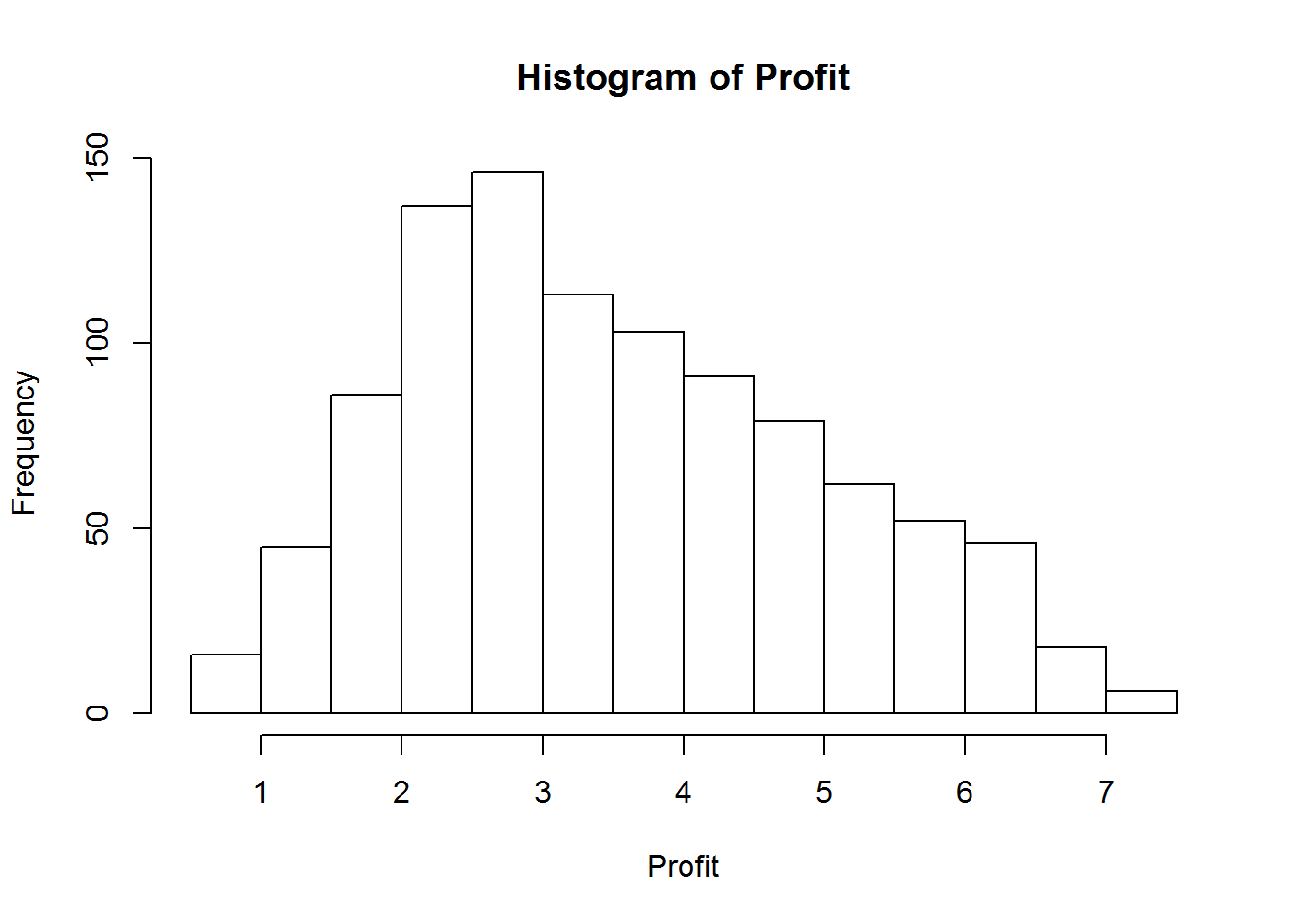R can be a powerful tool for Monte Carlo simulation. R’s primary strenth lies in statistical analysis, and has tons of functions which assist in using and exploring probability distributions, to include generating random numbers from these distributions. R can also assist in fitting data to a distribution (i.e. helping you determine what is the best distribution to use for a given data set). Additionally, you can use actual empirical data for Monte Carlo simulation, which is a concept we will explore in the next lesson. Below is a list of some of the distributions that are in R as well as the command that generates random numbers from that distribution.

Distribution Function
Triangle rtriangle (with the triangle package)
Beta rbeta
Binomial rbinom
Cauchy rcauchy
Chi-Square rchisq
Exponential rexp
F rf
Gamma rgamma
Geometric rgeom
Hypergeometric rhyper
Logistic rlogis
Log Normal rlnorm
Negative Binomial rnbinom
Normal rnorm
Poisson rpois
Student rt
Studentized Range rtukey
Uniform runif
Weibull rweibull
Wilcoxon Rank Sum Statistic rwilcox
Wilcoxon Signed Rank Statistic rsignrank

There are two primary ways to solve Monte Carlo problems in R. The first way is to place random numbers into some type of data structure such as a vector or matrix. The other way to go about Monte Carlo simulation in R is to conduct all of the computations for a each iteration inside a for loop. Today we will look at a simple Monte Carlo simulation problem, and discuss how we would tackle it with both a random variable vector as well as with a for loop.

# The Lemon Aid Stand

In the first day of class we discussed a problem in which an entrepreneurial young 5 year old decides to start a lemon aid stand in her cul de sac. Her equation for profit is $$Profit=(Price-Cost)*QtySold$$. The $$price=\ 0.10$$ and the $$cost=\ 0.05$$. The quantity sold per week is a triangular distribution with a low of 10, a high of 150, and a most likely value of 50. In Excel, we placed each of these random numbers into a single cell, and let the computer roll the dice for 1000 iterations, record the answer, and give it to us in a histogram graph of profit as well as the raw output.### Lemon Aid Profit with Vector

The first Monte Carlo method we could use in R to solve this problem would be to use a vector data structure. Remember, the only random variable we have it the quantity sold. If we created a vector of 1000 random numbers (from a triangular distribution with min=10, mode=50, and max=150), then we could multiply this vector by the marginal profit (price-cost) and arrive at a vector of 1000 profits. In R, this is accomplished like this:

## This code uses the triangle package.  Make sure you instll this
## first from the 'package' menu in the lower right screen in RStudio
library(triangle)
QtySold<-rtriangle(n=1000,a=10,b=150,c=50)

we have just created 1000 random triangular variables. If I wanted to look at the first 50 variables, I could do this with the following code:

QtySold[1:50]
##    47.18  58.75  52.89 110.18  79.59  91.21  72.73  50.43  29.09  22.82
##   52.24  60.39  72.60  87.25  52.00 114.36  81.71  70.42  40.71  42.78
##   69.61 113.08 101.96  69.11 103.24  43.56  81.36  58.89  50.00  52.69
##   69.92  25.48  65.26 113.34 112.04  79.25 115.93  65.22 109.72  52.47
##   60.08  57.47  76.92  58.70  70.75  98.72  68.43  27.36  53.88  38.99

These numbers all look like they’re coming from our designated random triangular distribution. Now I just need to multiply each of these number by my marginal profit in order to get a vector of total profit. In R, we do this with the following code.

Profit<-(0.10-0.05)*QtySold  ###Does it get any easier than this!!!!

Now we have a vector of 1000 profits. We can look at our first 50 values with the following code

Profit[1:50]
##   2.359 2.937 2.644 5.509 3.979 4.561 3.637 2.521 1.454 1.141 2.612
##  3.019 3.630 4.363 2.600 5.718 4.085 3.521 2.036 2.139 3.481 5.654
##  5.098 3.455 5.162 2.178 4.068 2.944 2.500 2.635 3.496 1.274 3.263
##  5.667 5.602 3.962 5.797 3.261 5.486 2.623 3.004 2.874 3.846 2.935
##  3.537 4.936 3.421 1.368 2.694 1.950

Now we need to plot a nice historgram and see how we can determine some statistics to answer the bosses questions. Let’s start by plotting a histogram. Remember, this is your answer in a stochastic problem.

hist(Profit)Now we need to look at the statistics. What was the minimum profit? Max profit? Mean Profit? The easiest way to answere this question is with the summary function.

summary(Profit)
##    Min. 1st Qu.  Median    Mean 3rd Qu.    Max.
##   0.611   2.400   3.320   3.520   4.560   7.190

If the ambitios 5-year old asked you what the probability is that she made more then $5, you would use the following code to answer that questions. This code is less intuitive than the previous code. In this code you are creating a new vector that only contains the values of profit that are greater than$5, and then measuring the length of that vector. This is best method of counting how many of the values are greater than $5. length(Profit[Profit>5]) ##Find how many iterations are over$5
##  183

This is how many of our 1000 iterations produced a profit that is greater than $5. If we want to calculate the probability, we simply divide this number by 1000: length(Profit[Profit>5])/1000 ##Calculate probability of being over$5
##  0.183

We see that it is close to 18%. Note that in R, it is very easy to increase our number of iterations (and much faster than Excel). If we wanted a more accurate number, we could use 100,000 random numbers. We do this in the following code and achieve a much more accurate answer:

QtySold<-rtriangle(n=100000,a=10,b=150,c=50)  ##Increase the number of Random Variable to 100,000
Profit<-(0.10-0.05)*QtySold   ##Recalculate Profit
length(Profit[Profit>5])/1000  ##Calculate probability of being over $5 ##  17.77 We see that the exact probability of being over$5 is 17.77%

### Lemon Aid Profit with Loop

You can also accomplish monte carlo simulation in R by using a loop. If we loop through each of our iterations, produce a random variable, conduct any necessary calculations, and store the answer in a location, then we have accomplished the same thing we discussed above. As problem get more comlicated, you are often forced to use a loop to run a monte carlo simulation. You could therefore solve the lemon aid monte carlo simulation problem with the following code:

Profit=NULL   ##This instantiates an object that we will use to store our answers
for(i in 1:1000) {  ##This is how you conduct a loop 1000 time in R
QtySold<-rtriangle(n=1,a=10,b=150,c=50) ## Calculate a SINGLE Triangular Random Variable
Profit[i]<-(0.10-0.05)*QtySold   ##Calculate the i'th profit
}

At the end of this, we have the same answer that we had before, as proved by the histogram plot below:

hist(Profit)## Homework

For homework, use R to solve the following problem:

A consumer electronics firm produces a line of battery rechargers for cell phones. The following distributions apply:

• Unit Price: triangular with a minimum of $18.95, most likely value of$24.95, and maximum of $26.95 • Unit Cost: uniform with minimum of$12.00 and maximum of $15.00 • Quantity Sold: 10,000-250*Unit price, plus a random term given by a normal distribution with a mean of 0 and a standard deviation of 10 • Fixed Costs: Normal with a mean of$30,000, and a standard deviation of \$5,000.
1. What is the expected profit?
2. What is the probability of a loss?
3. What is the maximum loss?